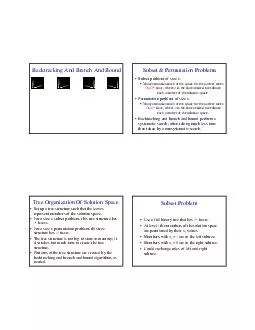# Backtracking And Branch And Bound Subset Permutation Problems Subset problem of size Nonsystematic search of the space for the answer ta kes Op time where p is the time needed to evaluate each membeEmbed code:

## Backtracking And Branch And Bound Subset Permutation Problems Subset problem of size Nonsystematic search of the space for the answer ta kes Op time where p is the time needed to evaluate each membe

Download Pdf - The PPT/PDF document "Backtracking And Branch And Bound Subset..." is the property of its rightful owner. Permission is granted to download and print the materials on this web site for personal, non-commercial use only, and to display it on your personal computer provided you do not modify the materials and that you retain all copyright notices contained in the materials. By downloading content from our website, you accept the terms of this agreement.

### Presentations text content in Backtracking And Branch And Bound Subset Permutation Problems Subset problem of size Nonsystematic search of the space for the answer ta kes Op time where p is the time needed to evaluate each membe

Page 1
Backtracking And Branch And Bound Subset & Permutation Problems Subset problem of size Nonsystematic search of the space for the answer ta kes O(p2 time, where p is the time needed to evaluate each member of the solution space. Permutation problem of size Nonsystematic search of the space for the answer ta kes O(pn!) time, where p is the time needed to evaluate each member of the solution space. Backtracking and branch and bound perform a systematic search; often taking much less time than taken by a nonsystematic search. Tree Organization Of Solution Space Set up a tree

structure such that the leaves represent members of the solution space. For a size subset problem, this tree structure has n leaves. For a size permutation problem, this tree structure has n! leaves. The tree structure is too big to store in memory; it also takes too much time to create the tree structure. Portions of the tree structure are created by the backtracking and branch and bound algorithms as needed. Subset Problem Use a full binary tree that has n leaves. At level i the members of the solution space are partitioned by their values. Members with = 1 are in the left subtree. Members

with = 0 are in the right subtree. Could exchange roles of left and right subtree.
Page 2
Subset Tree For n = 4 =1 = 0 =1 = 0 =1 = 0 =1 = 0 =1 =0 1110 1011 0111 0001 Permutation Problem Use a tree that has n! leaves. At level i the members of the solution space are partitioned by their values. Members (if any) with = 1 are in the first subtree. Members (if any) with = 2 are in the next subtree. And so on. Permutation Tree For n = 3 =1 =2 = 3 = 2 = 3 = 1 = 3 = 1 = 2 =3 =2 =3 =1 =2 =1 123 132 213 231 312 321 Backtracking Search the solution space tree in a depth- first manner. May be

done recursively or use a stack to retain the path from the root to the current node in the tree. The solution space tree exists only in your mind, not in the computer.
Page 3
Backtracking Depth-First Search =1 = 0 =1 = 0 =1 = 0 Backtracking Depth-First Search =1 = 0 =1 = 0 =1 = 0 Backtracking Depth-First Search =1 = 0 =1 = 0 =1 = 0 Backtracking Depth-First Search =1 = 0 =1 = 0 =1 = 0
Page 4
Backtracking Depth-First Search =1 = 0 =1 = 0 =1 = 0 O(2 ) Subet Sum & Bounding Functions =1 = 0 =1 = 0 =1 = 0 Each forward and backward move takes O(1) time. {10, 5, 2, 1}, c = 14

Bounding Functions When a node that represents a subset whose sum equals the desired sum , terminate. When a node that represents a subset whose sum exceeds the desired sum , backtrack. I.e., do not enter its subtrees, go back to parent node. Keep a variable r that gives you the sum of the numbers not yet considered. When you move to a right child, check if current subset sum + r >= c . If not, backtrack. Backtracking Space required is O(tree height) With effective bounding functions, large instances can often be solved. For some problems (e.g., 0/1 knapsack), the answer (or a very good

solution) may be found quickly but a lot of additional time is needed to complete the search of the tree. Run backtracking for as much time as is feasible and use best solution found up to that time.
Page 5
Branch And Bound Search the tree using a breadth-first search (FIFO branch and bound) Search the tree as in a bfs, but replace the FIFO queue with a stack (LIFO branch and bound) Replace the FIFO queue with a priority queue (least-cost (or max priority) branch and bound) . The priority of a node p in the queue is based on an estimate of the likelihood that the answer node is in

the subtree whose root is Branch And Bound Space required is O(number of leaves) For some problems, solutions are at different levels of the tree (e.g., 16 puzzle). 1 2 3 4 5 6 7 8 9 10 11 12 13 14 15 13 14 15 12 11 10 9 7 Branch And Bound FIFO branch and bound finds solution closest to roo t. Backtracking may never find a solution because tree depth is infinite (unless repeating configurations are eliminated). Least-cost branch and bound directs the search to parts of the space most likely to contain the answer. So it could perform better than backtracking.